# ML Aggarwal Class 10 Solutions for ICSE Maths Chapter 13 Similarity Ex 13.3

## ML Aggarwal Class 10 Solutions for ICSE Maths Chapter 13 Similarity Ex 13.3

ML Aggarwal Class 10 Solutions for ICSE Maths Chapter 13 Similarity Ex 13.3

Question 1.
Given that ∆s ABC and PQR are similar.
Find:
(i) The ratio of the area of ∆ABC to the area of ∆PQR if their corresponding sides are in the ratio 1 : 3.
(ii) the ratio of their corresponding sides if area of ∆ABC : area of ∆PQR = 25 : 36.
Solution:Question 2.
∆ABC ~ DEF. If area of ∆ABC = 9 sq. cm., area of ∆DEF =16 sq. cm and BC = 2.1 cm., find the length of EF.
Solution:Question 3.
∆ABC ~ ∆DEF. If BC = 3 cm, EF = 4 cm and area of ∆ABC = 54 sq. cm. Determine the area of ∆DEF.
Solution:Question 4.
The area of two similar triangles is 36 cm² and 25 cm². If an altitude of the first triangle is 2.4 cm, find the corresponding altitude of the other triangle.
Solution:Question 5.
(a) In the figure, (i) given below, PB and QA are perpendiculars to the line segment AB. If PO = 6 cm, QO = 9 cm and the area of ∆POB = 120 cm², find the area of ∆QOA. (2006)(b) In the figure (ii) given below, AB || DC. AO = 10 cm, OC = 5cm, AB = 6.5 cm and OD = 2.8 cm.
(i) Prove that ∆OAB ~ ∆OCD.
(ii) Find CD and OB.
(iii) Find the ratio of areas of ∆OAB and ∆OCD.Solution: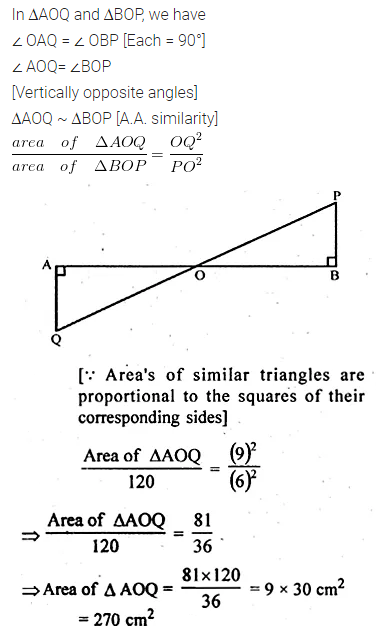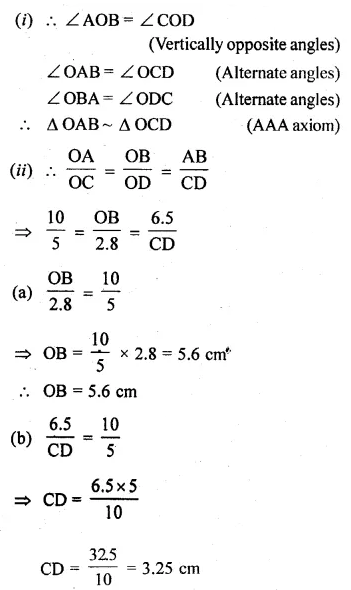Question 6.
(a) In the figure (i) given below, DE || BC. If DE = 6 cm, BC = 9 cm and area of ∆ADE = 28 sq. cm, find the area of ∆ABC.(b) In the figure (iii) given below, DE || BC and AD : DB = 1 : 2, find the ratio of the areas of ∆ADE and trapezium DBCE.Solution: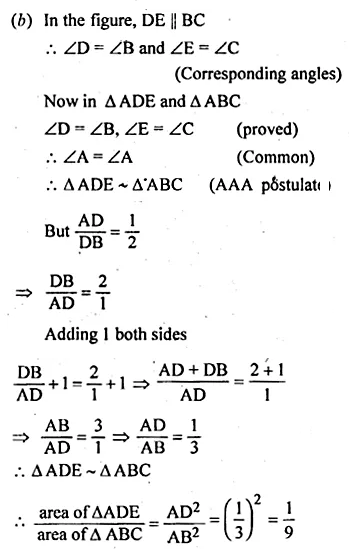Question 7.
In the given figure, DE || BC.
(i) Prove that ∆ADE and ∆ABC are similar.
(ii) Given that AD = $$\\ \frac { 1 }{ 2 }$$ BD, calculate DE if BC = 4.5 cm.(iii) If area of ∆ABC = 18 cm², find the area of trapezium DBCE
Solution: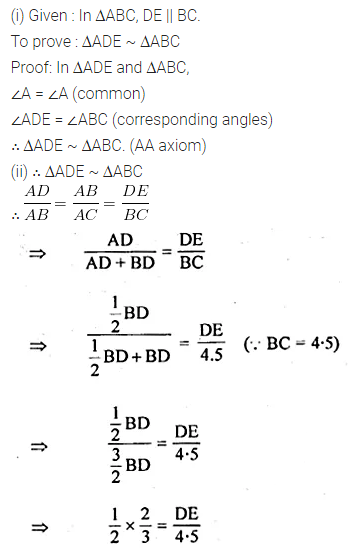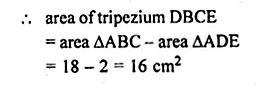Question 8.
In the given figure, AB and DE are perpendicular to BC.
(i) Prove that ∆ABC ~ ∆DEC
(ii) If AB = 6 cm: DE = 4 cm and AC = 15 cm, calculate CD.
(iii) Find the ratio of the area of ∆ABC : area of ∆DEC.Solution:Question 9.
In the adjoining figure, ABC is a triangle.
DE is parallel to BC and $$\frac { AD }{ DB } =\frac { 3 }{ 2 }$$
(i) Determine the ratios $$\frac { AD }{ AB }, \frac { DE }{ BC }$$
(ii) Prove that ∆DEF is similar to ∆CBF.
Hence, find $$\\ \frac { EF }{ FB }$$.
(iii) What is the ratio of the areas of ∆DEF and ∆CBF ? (2007)Solution:Question 10.
In ∆ABC, AP : PB = 2 : 3. PO is parallel to BC and is extended to Q so that CQ is parallel to BA. Find:
(i) area ∆APO : area ∆ABC.
(ii) area ∆APO : area ∆CQO. (2008)Solution:Question 11.
(a) In the figure (i) given below, ABCD is a trapezium in which AB || DC and AB = 2 CD. Determine the ratio of the areas of ∆AOB and ∆COD.
(b) In the figure (ii) given below, ABCD is a parallelogram. AM ⊥ DC and AN ⊥ CB. If AM = 6 cm, AN = 10 cm and the area of parallelogram ABCD is 45 cm², find
(i) AB
(ii) BC
(iii) area of ∆ADM : area of ∆ANB.
(c) In the figure (iii) given below, ABCD is a parallelogram. E is a point on AB, CE intersects the diagonal BD at O and EF || BC. If AE : EB = 2 : 3, find
(ii) area of ∆BEF : area of ∆ABD
(iii) area of ∆ABD : area of trap. AFED
(iv) area of ∆FEO : area of ∆OBC.Solution: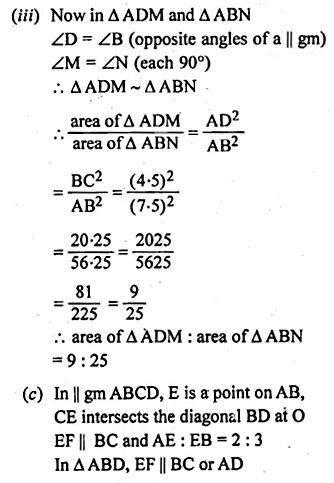Question 12.
In the adjoining figure, ABCD is a parallelogram. P is a point on BC such that BP : PC = 1 : 2 and DP produced meets AB produced at Q. If area of ∆CPQ = 20 cm², find(i) area of ∆BPQ.
(ii) area ∆CDP.
(iii) area of || gm ABCD.
Solution: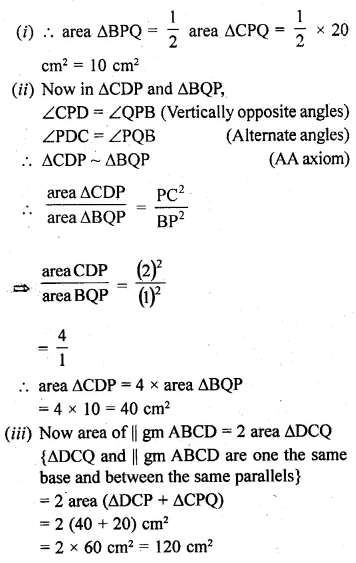Question 13.
(a) In the figure (i) given below, DE || BC and the ratio of the areas of ∆ADE and trapezium DBCE is 4 : 5. Find the ratio of DE : BC.(b) In the figure (ii) given below, AB || DC and AB = 2 DC. If AD = 3 cm, BC = 4 cm and AD, BC produced meet at E, find (i) ED (ii) BE (iii) area of ∆EDC : area of trapezium ABCD.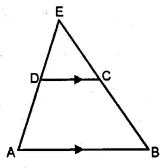Solution:Question 14.
(a) In the figure given below, ABCD is a trapezium in which DC is parallel to AB. If AB = 9 cm, DC = 6 cm and BB = 12 cm., find
(i) BP
(ii) the ratio of areas of ∆APB and ∆DPC.(b) In the figure given below, ∠ABC = ∠DAC and AB = 8 cm, AC = 4 cm, AD = 5 cm.
(i) Prove that ∆ACD is similar to ∆BCA
(ii) Find BC and CD
(iii) Find the area of ∆ACD : area of ∆ABC.Solution: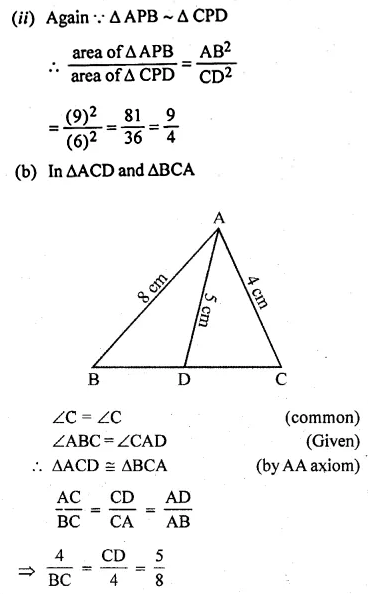Question 15.
ABC is a right angled triangle with ∠ABC = 90°. D is any point on AB and DE is perpendicular to AC. Prove that:
(ii) If AC = 13 cm, BC = 5 cm and AE = 4 cm. Find DE and AD.Solution: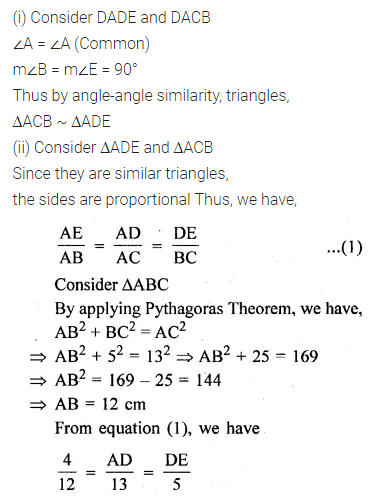Question 16.
Two isosceles triangles have equal vertical angles and their areas are in the ratio 7 : 16. Find the ratio of their corresponding height.
Solution:Question 17.
On a map is drawn to a scale of 1 : 250000, a triangular plot of land has the following measurements :
AB = 3 cm, BC = 4 cm and ∠ABC = 90°. Calculate
(i) the actual length of AB in km.
(ii) the area of the plot in sq. km:
Solution:Question 18.
On a map is drawn to a scale of 1 : 25000, a rectangular plot of land, ABCD has the following measurements AB = 12 cm and BG = 16 cm.
Calculate:
(i) the distance of a diagonal of the plot in km.
(ii) the area of the plot in sq. km.
Solution:Question 19.
The model of a building is constructed with scale factor 1 : 30.
(i) If the height of the model is 80 cm, find the actual height of the building in metres.
(ii) If the actual volume of a tank at the top of the building is 27 m³, find the volume of the tank on the top of the model. (2009)
Solution:Question 20.
A model of a ship is made to a scale of 1 : 200.
(i) If the length of the model is 4 m, find the length of the ship.
(ii) If the area of the deck of the ship is 160000 m², find the area of the deck of the model.
(iii) If the volume of the model is 200 litres, find the volume of the ship in m³. (100 litres = m³)
Solution: